## Method

Globally Consistent 3D LiDAR Mapping with GPU-accelerated GICP Matching Cost Factors [la] [GLIM]

Submitted on 8 Jun. 2021 06:58 by
Kenji Koide (National Institute of Advanced Industrial Science and Technology (AIST), Japan)

 Running time: 0.1 s Environment: GPU @ 2.5 Ghz (C/C++)

 Method Description: 3D LiDAR mapping system based on global matching cost minimization. It first removes dynamic objects and runs a frontend algorithm (e.g., MULLS), and then refines the estimated trajectory by minimizing the global registration error. The global optimization is performed on a middle-class GPU in real-time. Parameters: \voxel_resolution=0.5 Latex Bibtex: @Article{koide_ral2021, author = {Kenji Koide and Masashi Yokozuka and Shuji Oishi and Atsuhiko Banno}, journal = {{IEEE} Robotics and Automation Letters}, title = {Globally Consistent {3D} {LiDAR} Mapping with {GPU}-accelerated {GICP} Matching Cost Factors}, year = {2021}, doi = {10.1109/LRA.2021.3113043}, publisher = {{IEEE}}, }

## Detailed Results

From all test sequences (sequences 11-21), our benchmark computes translational and rotational errors for all possible subsequences of length (5,10,50,100,150,...,400) meters. Our evaluation ranks methods according to the average of those values, where errors are measured in percent (for translation) and in degrees per meter (for rotation). Details for different trajectory lengths and driving speeds can be found in the plots underneath. Furthermore, the first 5 test trajectories and error plots are shown below.

## Test Set AverageThis figure as: png eps pdf txt gnuplot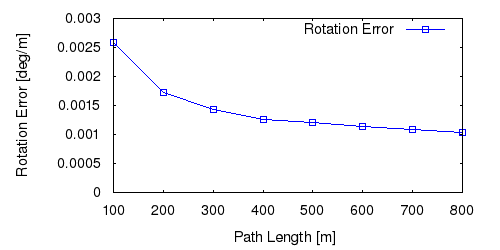This figure as: png eps pdf txt gnuplot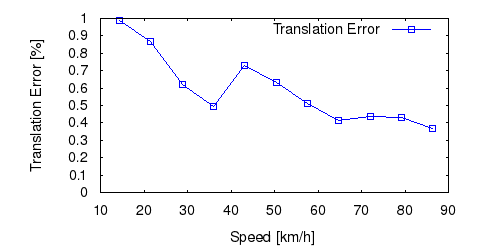This figure as: png eps pdf txt gnuplotThis figure as: png eps pdf txt gnuplot

## Sequence 11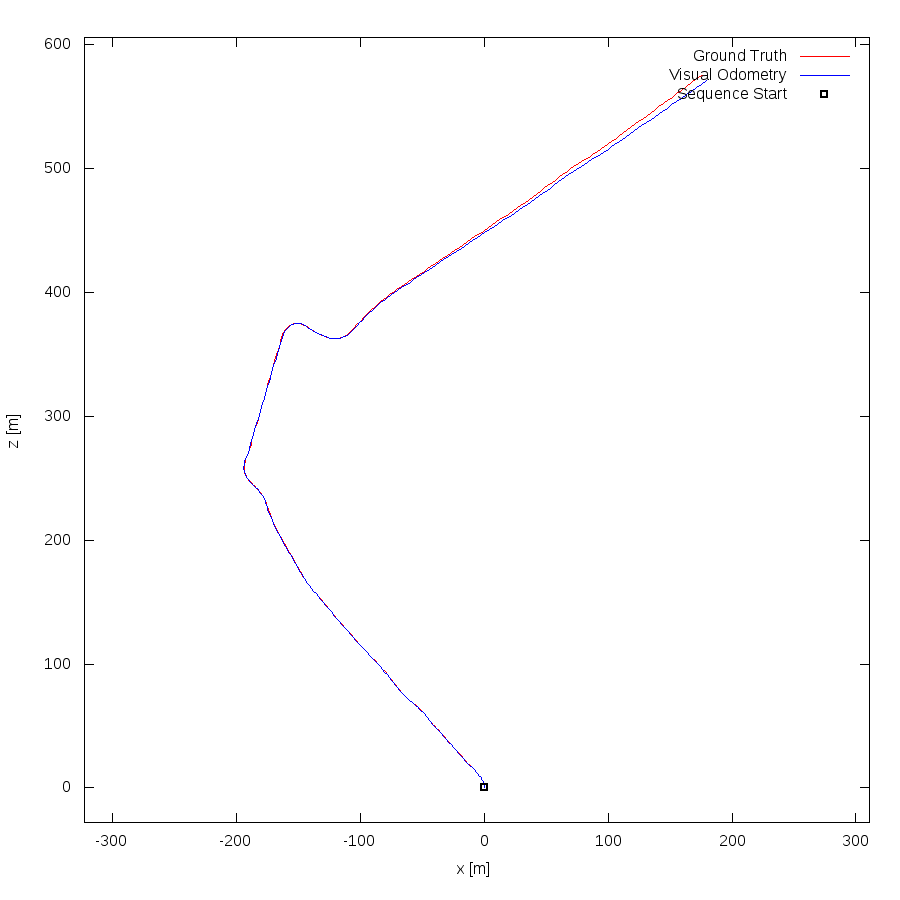This figure as: png eps pdf txt gnuplot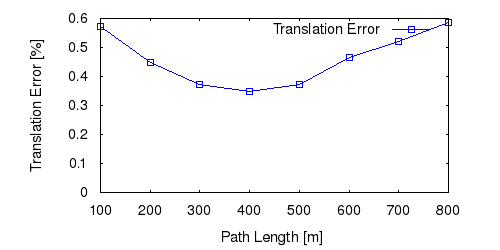This figure as: png eps pdf txt gnuplot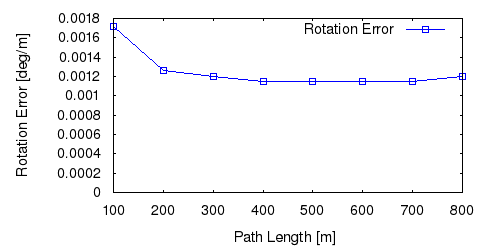This figure as: png eps pdf txt gnuplotThis figure as: png eps pdf txt gnuplot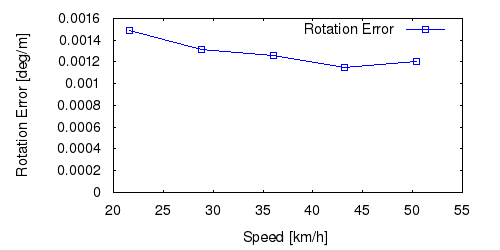This figure as: png eps pdf txt gnuplot

## Sequence 12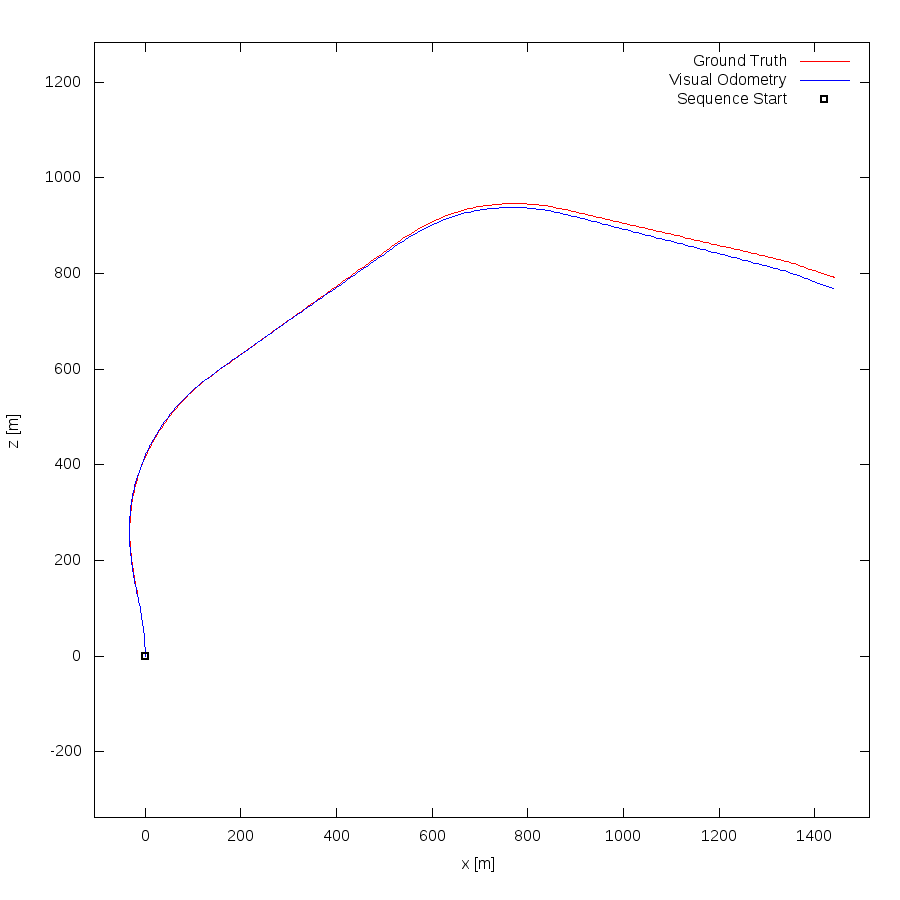This figure as: png eps pdf txt gnuplotThis figure as: png eps pdf txt gnuplot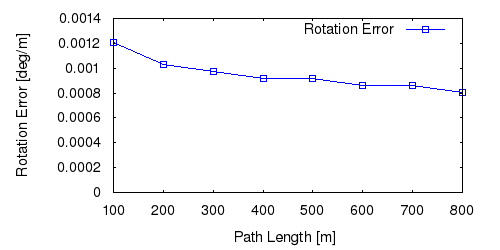This figure as: png eps pdf txt gnuplot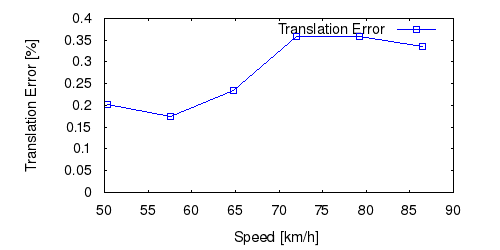This figure as: png eps pdf txt gnuplot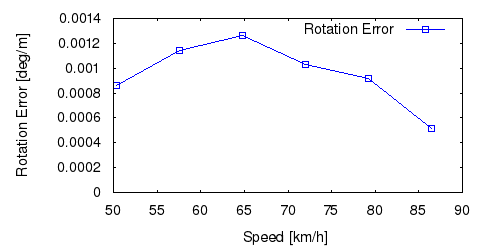This figure as: png eps pdf txt gnuplot

## Sequence 13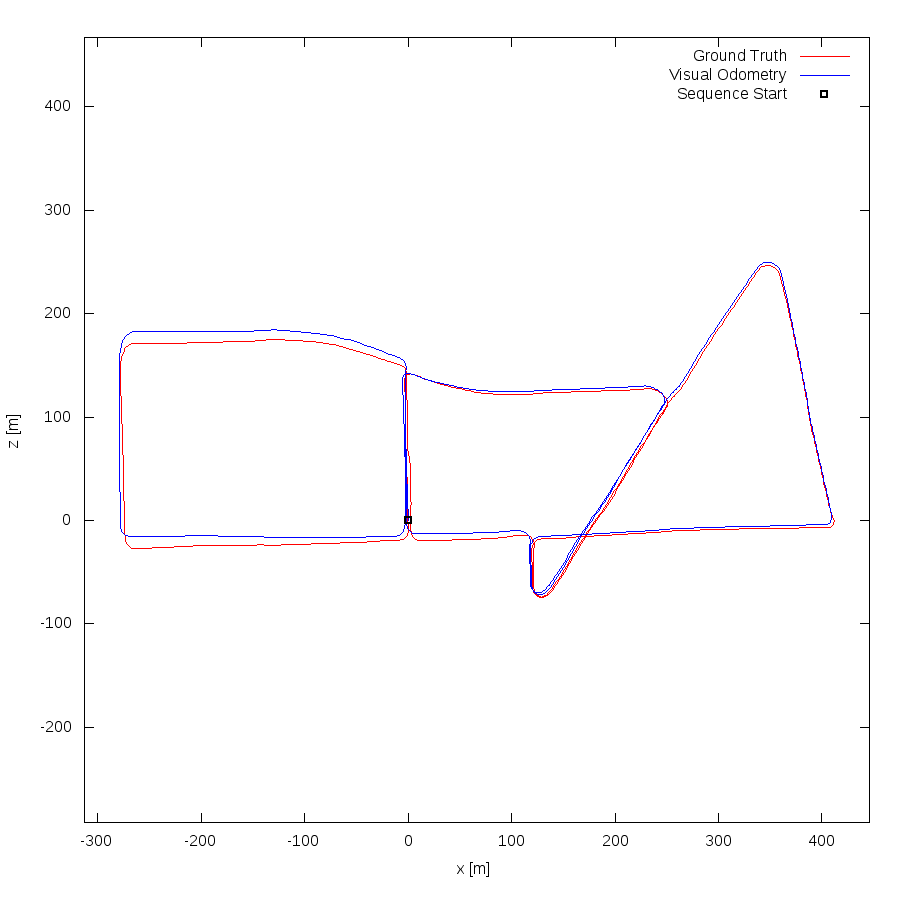This figure as: png eps pdf txt gnuplot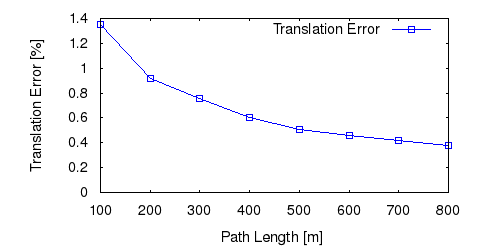This figure as: png eps pdf txt gnuplotThis figure as: png eps pdf txt gnuplot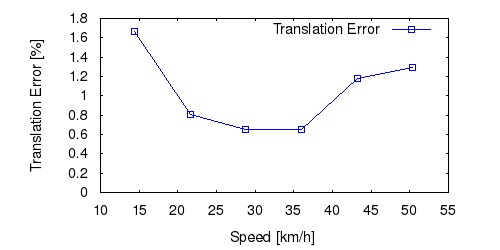This figure as: png eps pdf txt gnuplot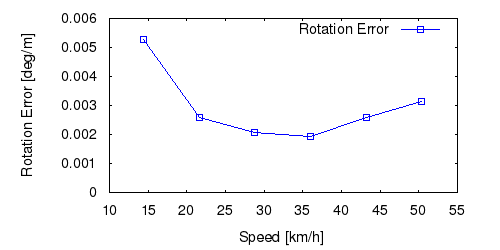This figure as: png eps pdf txt gnuplot

## Sequence 14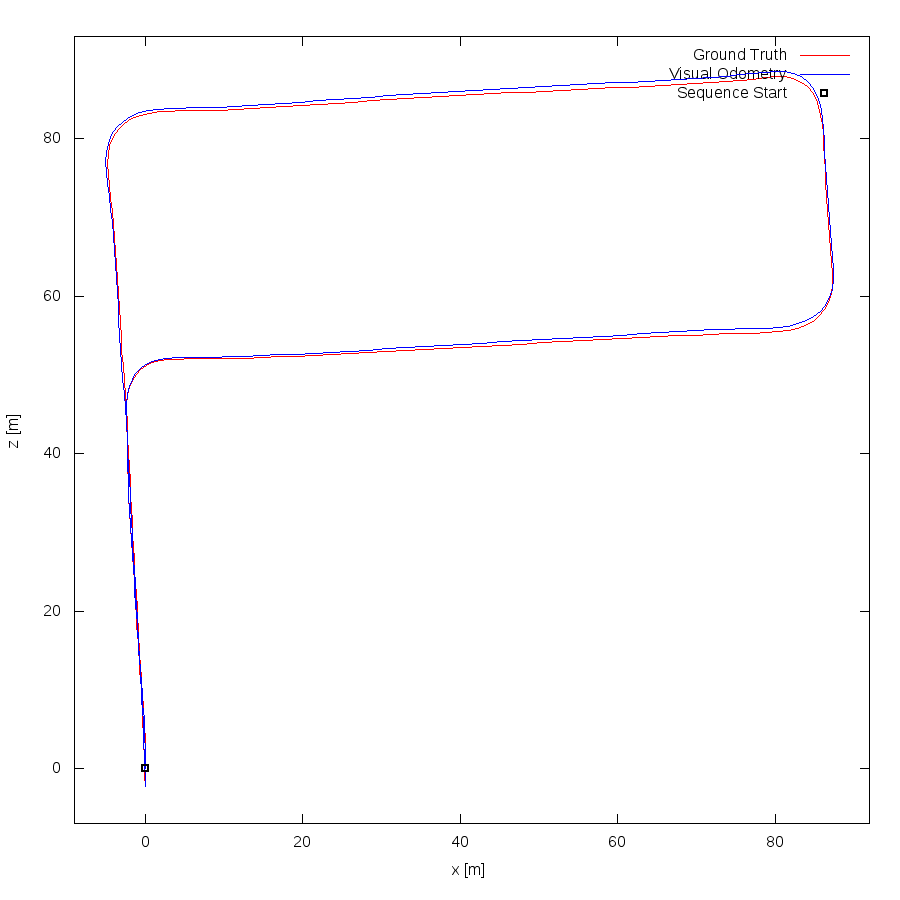This figure as: png eps pdf txt gnuplot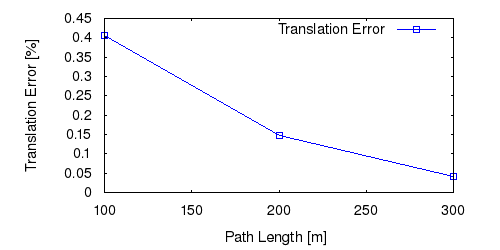This figure as: png eps pdf txt gnuplot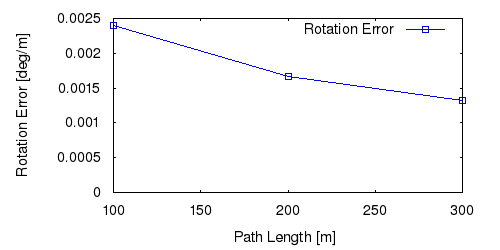This figure as: png eps pdf txt gnuplot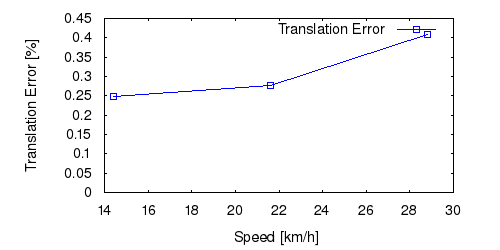This figure as: png eps pdf txt gnuplot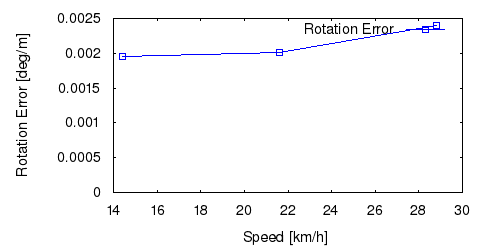This figure as: png eps pdf txt gnuplot

## Sequence 15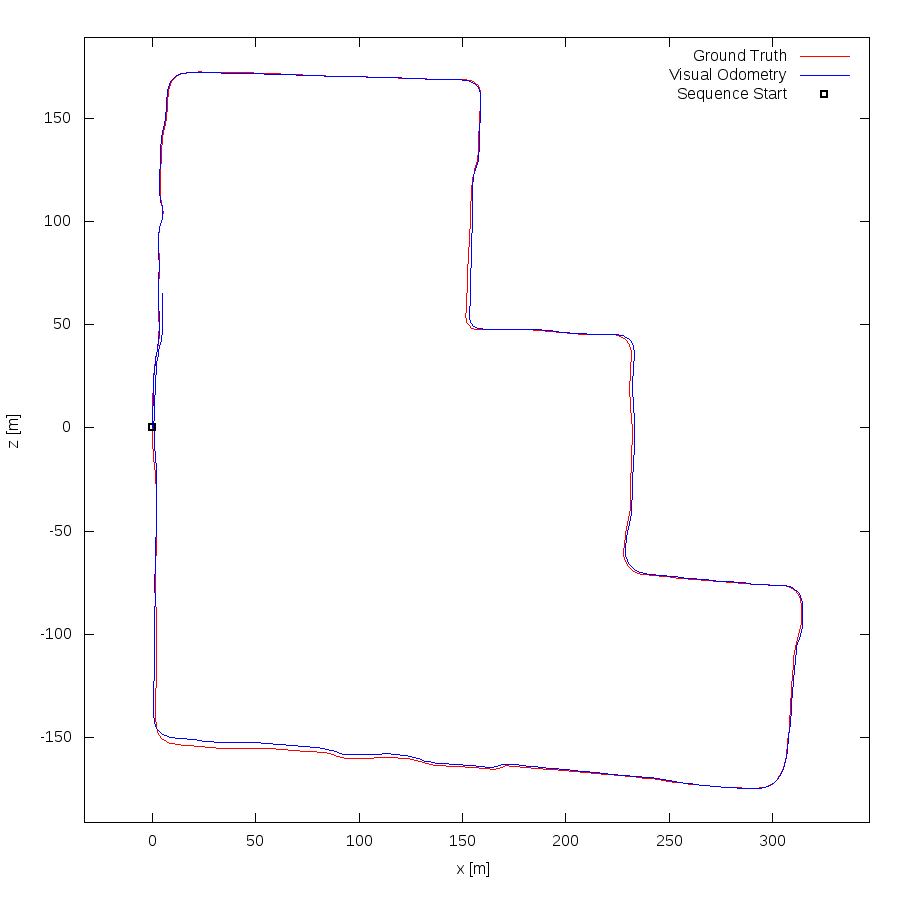This figure as: png eps pdf txt gnuplot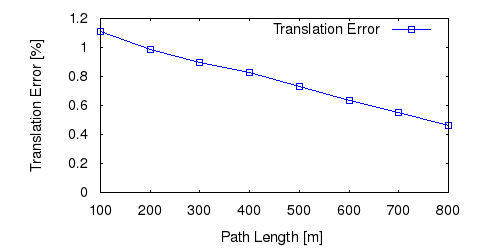This figure as: png eps pdf txt gnuplotThis figure as: png eps pdf txt gnuplot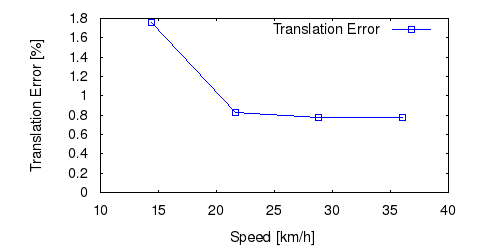This figure as: png eps pdf txt gnuplot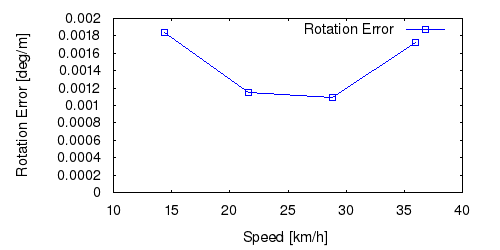This figure as: png eps pdf txt gnuplot